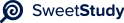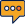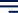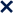•01 / 3100%A or N 80 and 106 68
345-6
Q.80 w2 + 30w + 81
Q.106 ac + xc + aw2 +xw2
Page 353
Q-68 10a2 â 27ab + 5b2
Because 10a^2 has factors 1a 10a and 2a 5a
5b^2 has factors 5b and b
We have four ways to solve
1a 5b 10a 5b 2a 5b 5a 5b
1a 1b 10a 1b 2a 1b 2a 1b
Factorisation means the given polynomial is written as product of factors. When we multiply the
factors â we get back the polynomial back.
Whenever there are two or more terms we try finding the GCF of all the terms, from variables we see
what is common to each term and the numbers are written as product of prime factors and then we
find GCF - then that factor is taken out of all the terms and we get a polynomial in factored form.
Quadratic polynomial can be a perfect square also. If its so, then the factorisation becomes very easy
and we can use the special formula of factorisation.
We use a method splitting the middle term, which gives us four terms and then we use grouping
method to find the factors.
We can do factorisa(on, using any of the three methods.
Spli-ng the middle term (ac method)
Comple(ng the Square
Pg. 345-6
80. w
2
+ 30w + 81 ( the 2âs are to the second power)
Arranging in proper form we get
w
2
+ 30w + 81
Spli-ng the middle term (ac method)
We get the two numbers as 27 and 3 which will give sum 30 and product 81
We get
w
2
+ 3w + 27w + 81
GCF of 6rst two terms is w and for last two terms is 27
w (w + 3) + 27(w + 3)
GcF of the two terms is w + 3
(w + 3)(w + 27) this is the factorisa(on106. ac + xc + aw
2
+xw
2
GCD of 6rst two terms is c and last two terms is w2
We get
c(a + x) + w
2
(a + x)
GCD of the two terms is (a + x0
We get
(a + x)( w
2
+c)
This is 6nal factorisa(on
Page 353
Q-68 10a
2
â 27ab + 5b
2
To be solved using trial and error method
10a
2
has factors a*10a and 2a*5a
5b
2
has factors b*5b
So we get four possible ways to factorize
Case I (a b)(10a 5b) Case II (2a b)(5a 5b)
Case III (a 5b)(10a b) Case IV (2a 5b)(5a b)
The case I and II is giving 5 as a common factor which is not in the original question so we reject
both cases.
Since last term in the expression is positive so we can have
(a â 5b)(10a â b) or (2a â 5b)(5a â b)
Checking both we get
(a â 5b)(10a â b) = a*10a â a*b â 5b*10a + 5b*b = 10a
2
â 16ab + 5b
2
(2a â 5b)(5a â b) = 2a*5a â 2a*b â 5b*5a + 5b*b = 10a
2
â 27ab + 5b
2
So correct factorization is 10a
2
â 16ab + 5b
2For the problems on page 345-6, factor the polynomials using whatever strategy seems appropriate.
State what methods you will use and then demonstrate the methods on your problems, explaining
the process as you go. Discuss any par(cular challenges those par(cular polynomials posed for the
factoring.
For the problem on page 353 make sure you use the âac methodâ regardless of what the bookâs
direc(ons say. Show the steps of this method in your work in a similar manner as how the book
shows it in examples.
Incorporate the following 6ve math vocabulary words into your discussion. Use bold font to
emphasize the words in your wri(ng (Do not write de6ni(ons for the words; use them appropriately
in sentences describing your math work.):
Factor
GCF
Prime factors
Perfect square
Grouping